# Electronics and Communication Engineering - Networks Analysis and Synthesis

1.

Assertion (A): Laplace transform of f(t) = e-at sin ωt is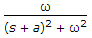Reason (R): If Laplace transform of f(t) is F(s), then Laplace transform of e-at f(t) is F(s + a).

 A. Both A and R are true and R is correct explanation of A B. Both A and R are true and R is not the correct explanation of A C. A is true but R is false D. A is false but R is true

Explanation:

No answer description available for this question. Let us discuss.

2.

A series RLC circuit is overdamped when

 A.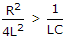B.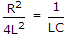C.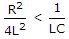D. R is infinite

Explanation:

No answer description available for this question. Let us discuss.

3.

The function (s + 2) (s + 4)/[(s + 1)(s + 3)]

 A. is a reactance function B. is an RC function C. may be a reactance function or an RC function D. may be RC function or an RL function

Explanation:

No answer description available for this question. Let us discuss.

4.

In figure, V1 = 40 V when R = 10 Ω, if R = 0, V2 will be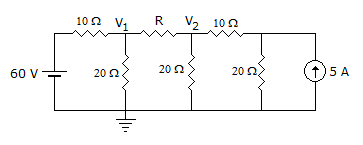A. 40 V B. 30 V C. 20 V D. 10 V

Explanation:

No answer description available for this question. Let us discuss.

5.

The series element of a band stop filter is

 A. capacitive B. inductive C. parallel combination of L and C D. series combination of L and C